Next: An example tridiagonal matrix Up: Poisson's equation Previous: Introduction

## 1-d problem with Dirichlet boundary conditions

As a simple test case, let us consider the solution of Poisson's equation in one dimension. Suppose that(113)

for, subject to the Dirichlet boundary conditions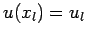and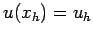.

As a first step, we divide the domaininto equal segments whose vertices are located at the grid-points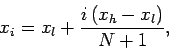(114)

for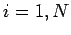. The boundaries,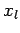and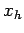, correspond to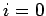and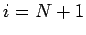, respectively.

Next, we discretize the differential term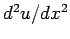on the grid-points. The most straightforward discretization is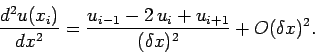(115)

Here,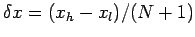, and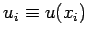. This type of discretization is termed a second-order, central difference scheme. It is second-order'' because the truncation error is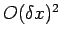, as can easily be demonstrated via Taylor expansion. Of course, an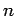th order scheme would have a truncation error which is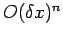. It is a central difference'' scheme because it is symmetric about the central grid-point,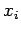. Our discretized version of Poisson's equation takes the form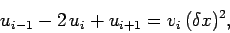(116)

for, where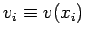. Furthermore,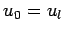and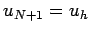.

It is helpful to regard the above set of discretized equations as a matrix equation. Let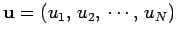be the vector of the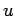-values, and let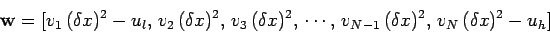(117)

be the vector of the source terms. The discretized equations can be written as: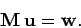(118)

The matrix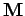takes the form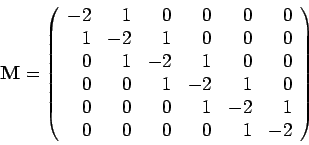(119)

for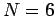. The generalization to othervalues is fairly obvious. Matrixis termed a tridiagonal matrix, since only those elements which lie on the three leading diagonals are non-zero.

The formal solution to Eq. (118) is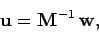(120)

where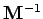is the inverse matrix to. Unfortunately, the most efficient general purpose algorithm for inverting an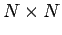matrix--namely, Gauss-Jordan elimination with partial pivoting--requires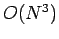arithmetic operations. It is fairly clear that this is a disastrous scaling for finite-difference solutions of Poisson's equation. Every time we doubled the resolution (i.e., doubled the number of grid-points) the required cpu time would increase by a factor of about eight. Consequently, adding a second dimension (which effectively requires the number of grid-points to be squared) would be prohibitively expensive in terms of cpu time. Fortunately, there is a well-known trick for inverting antridiagonal matrix which only requires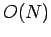arithmetic operations.

Consider a generaltridiagonal matrix equation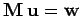. Let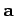,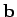, and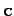be the vectors of the left, center and right diagonal elements of the matrix, respectively Note that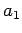and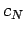are undefined, and can be conveniently set to zero. Our matrix equation can now be written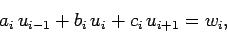(121)

for. Let us search for a solution of the form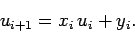(122)

Substitution into Eq. (121) yields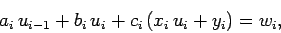(123)

which can be rearranged to give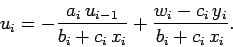(124)

However, if Eq. (122) is general then we can write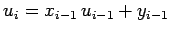. Comparison with the previous equation yields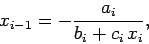(125)

and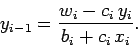(126)

We can now solve our tridiagonal matrix equation in two stages. In the first stage, we scan up the leading diagonal from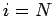to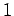using Eqs. (125) and (126). Thus,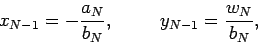(127)

since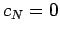. Furthermore,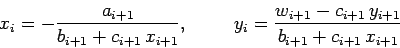(128)

for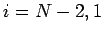. Finally,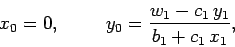(129)

since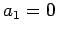. We have now defined all of theand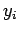. In the second stage, we scan down the leading diagonal fromto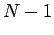using Eq. (122). Thus,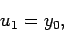(130)

since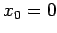, and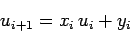(131)

for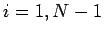. We have now inverted our tridiagonal matrix equation usingarithmetic operations.

Clearly, we can use the above algorithm to invert Eq. (118), with the source terms specified in Eq. (117), and the diagonals of matrixgiven by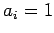for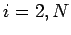, plus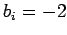for, and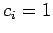for.Next: An example tridiagonal matrix Up: Poisson's equation Previous: Introduction
Richard Fitzpatrick 2006-03-29